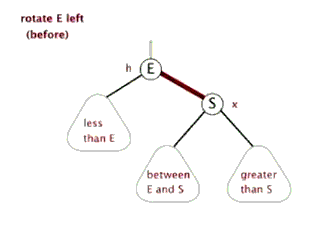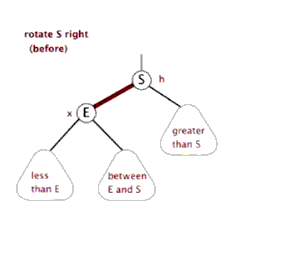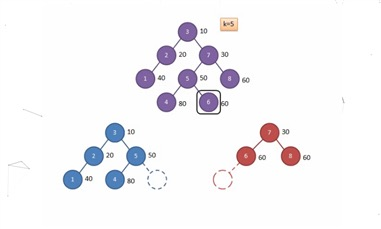nodeee 3月24日

# 关于平衡树

## Treap

Treap是一种平衡树的实现方法，在实际操作中并无太大作用。但是需要掌握它的思想。

Treap的核心思想是左旋和右旋。（以上图片摘自这个博客

（没写过，不会写，懒得写）

## fhq-Treap

kth操作没什么好多讲（当时查了三个小时的是谁啊？）

### 代码

#include<bits/stdc++.h>
using namespace std;
int n;
int sum,rnd,ch,siz;
int tot;
int root;
int new_node(int x){
tot++;
siz[tot]=1;
sum[tot]=x;
rnd[tot]=rand();
}
void update(int now){
siz[now]=siz[ch[now]]+siz[ch[now]]+1;
}
void split(int now,int a,int &x,int &y){
if(!now){
x=y=0;//
return ;
}
if(sum[now]<=a){//如果想要1~a,a+1~n的话就用<=
x=now;
split(ch[now],a,ch[now],y);
}else{
y=now;
split(ch[now],a,x,ch[now]);
}
update(now);
return ;
}
int merge(int x,int y){
if(!x||!y)return x+y;
if(rnd[x]<rnd[y]){
ch[x]=merge(ch[x],y);//有点难理解，建议自己画画看
update(x);
return x;
}else{
ch[y]=merge(x,ch[y]);
update(y);
return y;
}
}
int kth(int now,int a){
while(now){
if(siz[ch[now]]+1==a)return sum[now];
else if(siz[ch[now]]+1>a)now=ch[now];
else a-=siz[ch[now]]+1,now=ch[now];//注意a的更改是在now之前
}
}
int main(){
srand((unsigned)time(NULL));
int opt,a;
cin>>n;
for(int i=1;i<=n;i++){
cin>>opt>>a;
int x=0,y=0,z=0;
if(opt==1){
split(root,a,x,y);
root=merge(merge(x,new_node(a)),y);
}else if(opt==2){
split(root,a,x,y);
split(x,a-1,x,z);
z=merge(ch[z],ch[z]);
root=merge(merge(x,z),y);//这里顺序从小到大，请注意
}else if(opt==3){
split(root,a-1,x,y);
cout<<siz[x]+1<<endl;
root=merge(x,y);
}else if(opt==4){
cout<<kth(root,a)<<endl;
}else if(opt==5){
split(root,a-1,x,y);
cout<<kth(x,siz[x])<<endl;
root=merge(x,y);
}else if(opt==6){
split(root,a,x,y);
cout<<kth(y,1)<<endl;
root=merge(x,y);
}
}
return 0;
} 

split操作可参考下图（此图摘自洛谷日报）